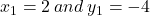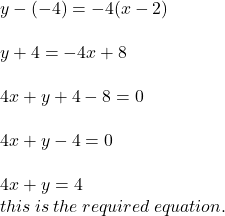## what is the equation standard form of through (2,-4))and the slope is -4

Question

what is the equation standard form of through (2,-4))and the slope is -4

in progress 0
6 months 2021-07-31T19:50:02+00:00 2 Answers 11 views 0

1. 4x + y = 4

First we can use the info to make a slope intercept form equation

y = -4x +4 (since slope is -4 and when x= 2, y = -4)

then change to slope intercept form (ax + by = c)

4x + y = 4Plugin the above equation we find: